Courses

# RD Sharma Solutions Ex-6.3, Factorization Of Polynomials, Class 9, Maths Class 9 Notes | EduRev

## Class 9 : RD Sharma Solutions Ex-6.3, Factorization Of Polynomials, Class 9, Maths Class 9 Notes | EduRev

The document RD Sharma Solutions Ex-6.3, Factorization Of Polynomials, Class 9, Maths Class 9 Notes | EduRev is a part of the Class 9 Course RD Sharma Solutions for Class 9 Mathematics.
All you need of Class 9 at this link: Class 9

In each of the following , using the remainder theorem, find the remainder when f(x) is divided by  g(x) and verify the by actual division : (1 – 8)

Q1. f(x) = x3+4x2–3x+10 , g(x) = x + 4

Sol :

Here,  f(x) = x3+4x2–3x+10

g(x) = x + 4

from, the remainder theorem when f(x) is divided by g(x) = x – (-4)  the remainder will be equal to f(-4)

Let , g(x) = 0

⇒ x + 4 = 0

⇒ x = -4

Substitute the value of x in f(x)

f(-4) = (−4)3+4(−4)2–3(−4)+10

= -64 + ( 4 * 16) + 12 + 10

= -64 + 64 + 12 + 10

= 12 + 10

= 22

Therefore, the remainder is 22

Q2. f(x) = 4x4–3x3–2x2+x–7 , g(x) = x – 1

Sol :

Here, f(x) = 4x4–3x3–2x2+x–7

g(x) = x – 1

from, the remainder theorem when f(x) is divided by g(x) = x – (-1) the remainder will be equal to f(1)

Let , g(x) = 0

⇒ x – 1 = 0

⇒ x = 1

Substitute the value of x in f(x)

f(1) = 4(1)4–3(1)3–2(1)2+1–7

= 4 – 3 – 2 + 1 – 7

= 5 – 12

= -7

Therefore, the remainder is 7

Q3. f(x) = 2x4–6x3+2x2–x+2 , g(x) = x + 2

Sol :

Here, f(x) = 2x4–6x3+2x2–x+2

g(x) = x + 2

from, the remainder theorem when f(x) is divided by g(x) = x – (-2) the remainder will be equal to f(-2)

Let , g(x) = 0

⇒ x + 2 = 0

⇒ x = -2

Substitute the value of x in f(x)

f(-2) = 2(−2)4–6(−2)3+2(−2)2–(−2)+2

= (2 * 16) – (6 * (-8)) + (2 * 4) + 2 + 2

= 32 + 48 + 8 + 2 + 2

= 92

Therefore, the remainder is 92

Q4. f(x) = 4x3–12x2+14x–3 , g(x) = 2x – 1

Sol:

Here, f(x) = 4x3–12x2+14x–3

g(x) = 2x – 1

from, the remainder theorem when f(x) is divided by g(x) =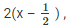the remainder is equal to f(1/2)

Let , g(x) = 0

⇒ 2x – 1 = 0

⇒ 2x = 1

⇒ x = 1/2

Substitute the value of x in f(x)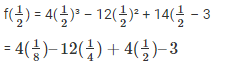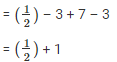Taking L.C.M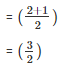Therefore, the remainder is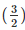Q5. f(x) = x3–6x2+2x–4 , g(x) = 1 – 2x

Sol :

Here, f(x) = x3–6x2+2x–4

g(x) = 1 – 2x

from, the remainder theorem when f(x) is divided by g(x) = -the remainder is equal to f(1/2)

Let , g(x) = 0

⇒ 1 – 2x  = 0

⇒ -2x = -1

⇒ 2x = 1

⇒ x = 1/2

Substitute the value of x in f(x)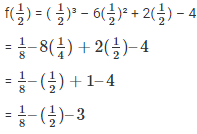Taking L.C.M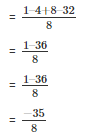Therefore, the remainder is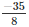Q6. f(x) = x4–3x2+4 , g(x) = x – 2

Sol :

Here, f(x) = x4–3x2+4

g(x) = x – 2

from, the remainder theorem when f(x) is divided by g(x) = x – 2 the remainder will be equal to f(2)

let , g(x) = 0

⇒ x – 2 = 0

⇒ x = 2

Substitute the value of x in f(x)

f(2) = 24–3(2)2+4

= 16 – (3* 4) + 4

= 16 – 12 + 4

= 20 – 12

= 8

Therefore, the remainder is 8

Q7. f(x) = 9x3–3x2+x–5 , g(x)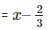Sol :

Here, f(x) = 9x3–3x2+x–5

g(x) =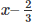from, the remainder theorem when f(x) is divided by g(x) =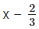the remainder will be equal to f(1/2) substitute the value of x in f(x)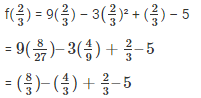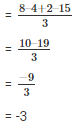Therefore, the remainder is -3

Q8.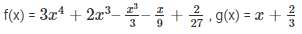Sol :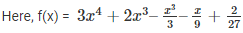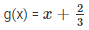from remainder theorem when f(x) is divided by g(x)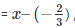the remainder is equal to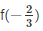substitute the value of x in f(x)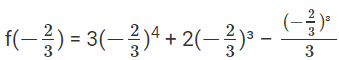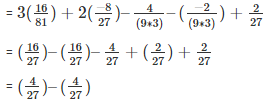= 0

Therefore, the remainder is 0

Q9. If the polynomial 2x3+ax2+3x–5 and x3+x2–4x+a leave the same remainder when divided by x – 2 , Find the value of a

Sol :

Given , the polymials are

f(x) = 2x3+ax2+3x–5

p(x) = x3+x2–4x+a

The remainders are f(2) and p(2) when f(x) and p(x) are divided by x – 2

We know that,

f(2) = p(2)   (given in problem)

we need to  calculate f(2) and p(2)

for, f(2)

substitute (x = 2) in f(x)

f(2) =  2(2)3+a(2)2+3(2)–5

= (2 * 8) + a4 + 6 – 5

= 16 + 4a + 1

= 4a + 17 ——- 1

for, p(2)

substitute (x = 2) in p(x)

p(2) = 23+22–4(2)+a

= 8 + 4 – 8 + a

= 4 + a  ——— 2

Since, f(2) = p(2)

Equate eqn 1 and 2

⇒ 4a + 17 = 4 + a

⇒ 4a – a = 4 – 17

⇒ 3a = -13

⇒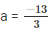Q10. If polynomials ax3+3x2–3 and 2x3–5x+a when divided by (x – 4) leave the remainders as Rand R2 respectively. Find the values of a in each of the following cases, if

1. R1 = R2
2. R1+R2 = 0
3. 2R1–R2 = 0

Sol :

Here, the polynomials are

f(x) = ax3+3x2–3

p(x) = 2x3–5x+a

let,

R1 is the remainder when f(x) is divided by x – 4

⇒  R1 = f(4)

⇒ R1 = a(4)3+3(4)2–3

= 64a + 48 – 3

= 64a + 45               ——— 1

Now , let

R2 is the remainder when p(x) is divided by x – 4

⇒ R2 = p(4)

⇒ R2 = 2(4)3–5(4)+a

= 128 – 20 + a

= 108 + a    ——– 2

1. Given , R1 = R2

⇒ 64a + 45 = 108 + a

⇒ 63a = 63

⇒ a = 1

2. Given, R1+R2 = 0

⇒ 64a + 45 + 108 + a = 0

⇒ 65a + 153 = 0

⇒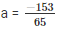3.Given, 2R1–R2 = 0

⇒ 2(64a + 45) – 108 – a = 0

⇒ 128a + 90 – 108 – a = 0

⇒ 127a – 18 = 0

⇒  a = 18/127

Q11. If the polynomials ax3+3x2–13 and 2x3–5x+a when divided by (x – 2) leave the same remainder, Find the value of a

Sol :

Here , the polynomials are

f(x) =  ax3+3x2–13

p(x) = 2x3–5x+a

equate , x – 2 = 0

x = 2

substitute the value of x in f(x) and p(x)

f(2) = (2)3+3(2)2–13

= 8a + 12 – 13

= 8a – 1            ———- 1

p(2) = 2(2)3–5(2)+a

= 16 – 10 + a

= 6 + a               ———– 2

f(2) = p(2)

⇒ 8a – 1 = 6 + a

⇒ 8a – a = 6 + 1

⇒ 7a = 7

⇒ a = 1

The value of a = 1

Q12. Find the remainder when x3+3x3+3x+1 is divided by,

1. x + 1
2.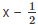3. x
4. x + π
5. 5 + 2x

Sol :

Here, f(x) =  x3+3x2+3x+1

by remainder theorem

1. ⇒ x + 1 = 0

⇒ x = -1

substitute the value of x in f(x)

f(-1) = (−1)3+3(−1)2+3(−1)+1

= -1  + 3 – 3 + 1

= 0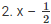Here, f(x) =  x3+3x2+3x+1

By remainder theorem

⇒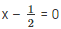⇒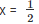substitute the value of x in f(x)= 27/8

3. x

Sol :

Here, f(x) =  x3+3x2+3x+1

by remainder theorem

⇒ x = 0

substitute the value of x in f(x)

f(0) =  03+3(0)2+3(0)+1

= 0 + 0 + 0 + 1

= 1

4. x + π

Sol :

Here, f(x) =  x3+3x2+3x+1

by remainder theorem

⇒ x + π = 0

⇒ x =  -π

Substitute the value of x in f(x)

f(-π) =  (−π)3+3(−π)2+3(−π)+1

= –(π)3+3(π)2–3(π)+1

5. 5 + 2x

Sol :

Here, f(x) =  x3+3x2+3x+1

by remainder theorem

5 + 2x = 0

2x = -5

x = -5/2

substitute the value of x in f(x)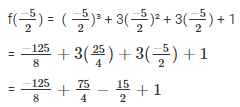Taking L.C.M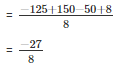Offer running on EduRev: Apply code STAYHOME200 to get INR 200 off on our premium plan EduRev Infinity!

91 docs

,

,

,

,

,

,

,

,

,

,

,

,

,

,

,

,

,

,

,

,

,

,

,

,

,

,

,

,

,

,

;